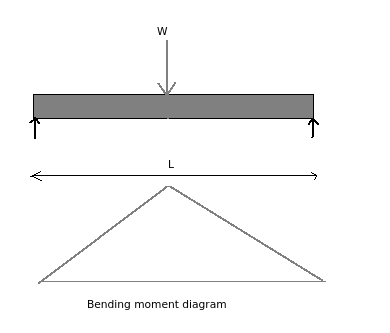# Numerical - Bending moment, given simply supported beam?

Q.  What is the maximum bending moment acting on simply supported beam shown below?
- Published on 21 Sep 15a. 2000 Nm
b. 3000 Nm
c. 4000 Nm
d. 6000 Nm

#### Discussion

• Sravanthi   -Posted on 24 Nov 15- Bending moment diagram for simply supported beam is as shown above. The formula used to calculate bending moment at central point load is given as:

M = W/2 x (L/2) = WL/4

Given: Load (W) = 600 N, Length of beam (L) = 20 m

Formula: Bending moment (M) = WL/4

Solution:

Substituting the given values, we get

Bending moment (M) = WL/4

= (600 x 20) / 4

= 3000 Nm

Bending moment (M) for simply supported beam is 3000 Nm.

## ➨ Post your comment / Share knowledgeEnter the code shown above:

(Note: If you cannot read the numbers in the above image, reload the page to generate a new one.)